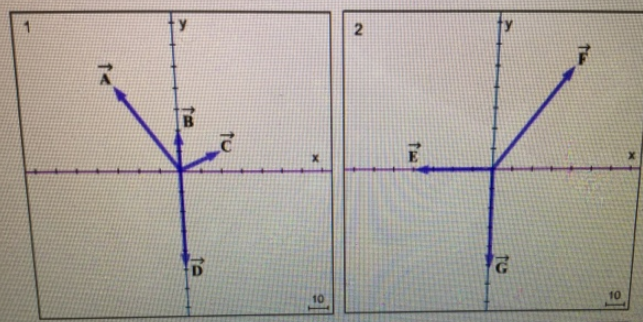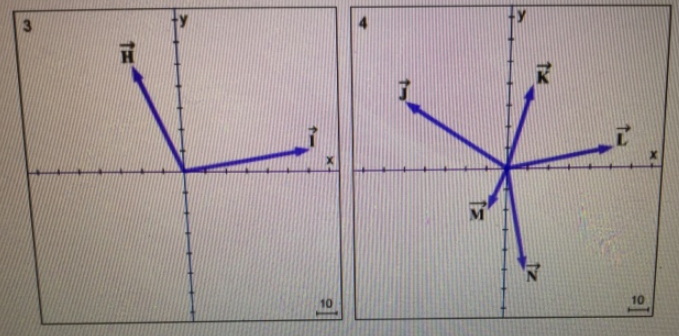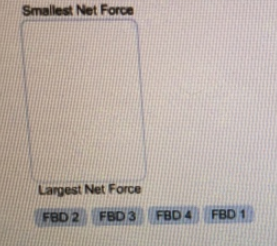# Problem: Analyze the Freebody diagram, and rank them according to the magnitude of the net force.

###### FREE Expert Solution

The net force is the sum of all the forces acting.

Vector magnitude:

$\overline{)\mathbf{|}\stackrel{\mathbf{⇀}}{\mathbit{A}}\mathbf{|}{\mathbf{=}}\sqrt{{{\mathbit{A}}_{\mathbit{x}}}^{\mathbf{2}}\mathbf{+}{{\mathbit{A}}_{\mathbit{y}}}^{\mathbf{2}}}}$

FBD 1

80% (190 ratings)###### Problem Details

Analyze the Freebody diagram, and rank them according to the magnitude of the net force.Frequently Asked Questions

What scientific concept do you need to know in order to solve this problem?

Our tutors have indicated that to solve this problem you will need to apply the Vector Composition & Decomposition concept. You can view video lessons to learn Vector Composition & Decomposition. Or if you need more Vector Composition & Decomposition practice, you can also practice Vector Composition & Decomposition practice problems.

What professor is this problem relevant for?

Based on our data, we think this problem is relevant for Professor DeJonghe's class at UIC.